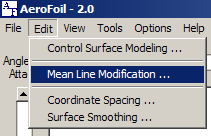## Mean Line ModificationModification of a mean line is an older method of altering an airfoil in an attempt to change its characteristics. The current airfoil data is de-composed into a thickness distribution and a mean line. The calculated mean line may then modified with the NACA mean line equations, or the thickness profile may be combined with an entirely new mean line. The mean line and thickness distribution is combined to give the airfoil shape in the same manner as with the NACA 4, 5, and 6 digit series airfoils.

A mathematical model known as the theory of thin wing sections was first developed in the 20’s and refined throughout the 30’s. Researchers of the era discovered that most of the characteristics of airfoils could be determined from the shape of the mean line. In this model, a series of small vortices were placed along a line that represented an airfoil’s mean line. The strength of each vortex is adjusted mathematically until the resulting airflow is a streamline along the surface of the line. The mathematical model developed by Theodorsen is used to estimate the angle of zero lift, Pitch Coefficient, and design lift coefficient that will result from the mean line.

The calculation must first ensure that there are an identical number of data points on both the top and bottom surfaces. In a modern airfoil, there are typically more data points defining the top surface than the bottom. The de-composition routine adds coordinates as necessary to the bottom surface and recalculates their location using a spline fit.

The user may specify which type of mean line equation will be used. The default is a NACA 6 digit type. The NACA 4 digit type is also available.